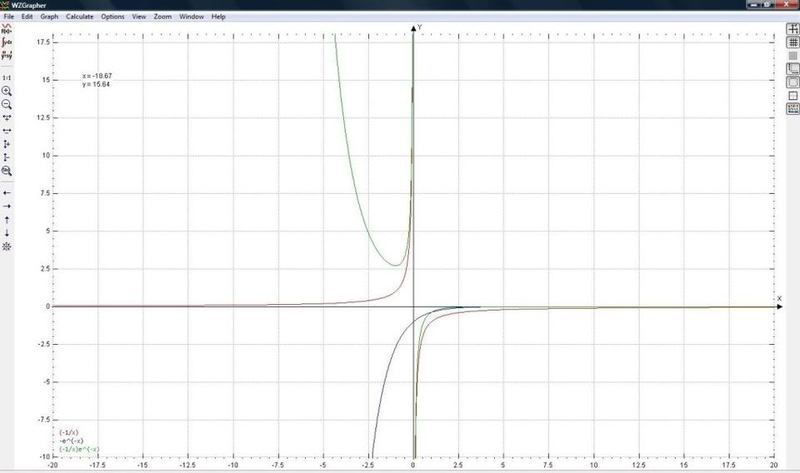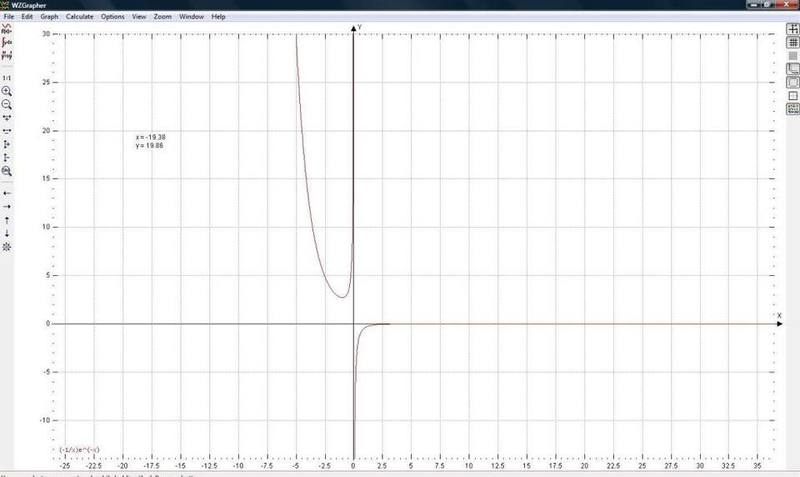# Yukawa Potential

Hart

## Homework Statement

The Yukawa potential is given by:

$$V_{\gamma}(r) = -\frac{q^{2}}{4\pi \epsilon_{0}r}e^{-\gamma r}$$

Where $$\gamma$$ is a constant. This describes a screened Coulomb potential.

I. Sketch the radial dependance of this potential.

II. State the radial Schodinger equation for this potential.

III. Assume that $\gamma$ is a small parameter. Use first order perturbation theory to estimate the energy shift of the ground state of the hydrogen atom when the Coulomb potential is replaced by the Yukawa potential. Hint: The perturbation is:

$$\omega = V_{\gamma}-V_{0}$$.

## Homework Equations

Within the question statement / answers.

## The Attempt at a Solution

I don’t seem to be able to find much useful information about this potential which I can understand and/or use to try and answer this question.

I. Obviously I know that it’s hard to show what it looks like over these forums, but any description of what it looks like would be great.. I don’t know.

II. Help and advice needed!

III. Again, help and advice needed!

.. sorry I don't have really any input at the moment, but I really don't get what's going on here and havn't been able to find much info elsewhere.

## Answers and Replies

nickjer
I) Just plot it in a graphing calculator or some software. It will look like:

$$\frac{-1}{r}e^{-r}$$

2) Just write out the Schrodinger equation in spherical coords using this potential for V(r)

3) Expand that perturbation to first order in lambda using a Taylor expansion. Then operate on this perturbation with the ground state:

$$E_0^{(1)} = <0|V_{\lambda}-V_0|0>$$

where |0> is the ground state of the hydrogen atom.

Hart
1. I don't have a graphical calculator and I havn't really managed to find any good free graphing software from online searches.. any useful links or advice?

2. So the Schrodinger equation in spherical polar coordinates:

$$E \psi \left(r, \theta, \phi \right) = \left[-\frac{\hbar^{2}}{2mr^{2}}\frac{\partial}{\partial r}\left(r^{2}\frac{\partial}{\partial r}\right) - \frac{\hbar^{2}}{2mr^{2}}\left(\frac{1}{sin \theta}\frac{\partial}{\partial \theta}\left(sin \theta \frac{\partial}{\partial \theta}\right) + \frac{1}{sin^{2}\theta}\frac{\partial^{2}}{\partial \phi^{2}}\right) + V(r)\right] \psi \left( r, \theta, \phi \right)$$

Hence with input of the potential defined:

$$E \psi \left(r, \theta, \phi \right) = \left[-\frac{\hbar^{2}}{2mr^{2}}\frac{\partial}{\partial r}\left(r^{2}\frac{\partial}{\partial r}\right) - \frac{\hbar^{2}}{2mr^{2}}\left(\frac{1}{sin \theta}\frac{\partial}{\partial \theta}\left(sin \theta \frac{\partial}{\partial \theta}\right) + \frac{1}{sin^{2}\theta}\frac{\partial^{2}}{\partial \phi^{2}}\right) + -\frac{q^{2}}{4\pi \epsilon_{0}r}e^{-\gamma r}\right] \psi \left( r, \theta, \phi \right)$$

3. I'm not sure how to do the Taylor expansion / perturbation thing :|

Hart
.. still can't figure out how to do part 3 of this questionThis is as far as I've got:

$$E_{n}^{1} = E_{0}' - E_{0} \approx \left< \psi_{n}^{0}|\omega|\psi_{n}^{0}\right>$$

so..

$$E_{n}^{1} = E_{0}' - E_{0} \approx \left< \psi_{n}^{0}|V_{\gamma}-V_{0}|\psi_{n}^{0}\right>$$

.. and then I need to do some sort of Taylor expansion thing with the perturbation?!

Really don't get this :grumpy:

nickjer
What is the Taylor Expansion for an exponential?

nickjer
Also when they ask for the radial Schrodinger Eqn, I think they only want it in terms of 'r' and not theta and phi. You can separate out theta and phi using spherical harmonics.

Homework Helper
Gold Member
1. I don't have a graphical calculator and I haven't really managed to find any good free graphing software from online searches.. any useful links or advice?

At large $r$, $e^{-r}$ falls off much faster than any polynomial of $r$, so you would expect it to look a lot like an inverted (because of the negative sign in front) decaying exponential there.

For small $r$, $e^{-r}\approx 1-r$ so you would expect it to (again inverted) decay like $1/r$ from $-\infty$ there.

In between the two extremes, you would expect it to decay slightly faster than an exponential, from a large negative value towards zero.

As for graphing software, gnuplot should be more than enough. I suggest you do three plots and superimpose them. Plot $-\frac{1}{r}$, $-e^{-r}$ and $-\frac{1}{r}e^{-r}$ together so you can compare their behavior at large and small $r$. It is also worth noting that $\frac{1}{r}e^{-r}=1$ at $r\approx 0.567$, so you may want to adjust the range of at least one of your graphs to examine the behavior near this point.

Hart
Taylor Expansion for an Exponential:

Isn't it this..

$$e^{x} = \sum_{n=0}^{\infty}\frac{x^{n}}{n!} = 1 + x + \frac{x^{2}}{2!} + \frac{x^{3}}{3!}+ ...$$

??!

If so, presumably then have to put the function:

$$\frac{-1}{r}e^{-r}$$

into it? where x = r?

.. oh, so you think it should be this instead:

$$ER = -\frac{\hbar^{2}}{2m_{e}}\frac{1}{r^{2}}\frac{d}{dr}\left(r^{2}\frac{dR}{dr}\right) + \left[V(r) + \frac{l(l+1)\hbar}^{2}{2m_{e}r^{2}}\right]R$$

then input given potential:

$$V_{\gamma}(r) = -\frac{q^{2}}{4\pi \epsilon_{0}r}e^{-\gamma r}$$

hence:

$$ER = -\frac{\hbar^{2}}{2m_{e}}\frac{1}{r^{2}}\frac{d}{dr}\left(r^{2}\frac{dR}{dr}\right) + \left[-\frac{q^{2}}{4\pi \epsilon_{0}r}e^{-\gamma r} + \frac{l(l+1)\hbar^{2}}{2m_{e}r^{2}}\right]R$$

?!

Yukawa Plot:

I plotted the three functions as suggested, and using the software suggested, this is a plot of them:

(nb1: apologies, can't figure out how to make the images smaller.)
(nb2: had to use x as variable not r, it wouldn't let me plot it otherwise)So the Yukawa Potential should look like this:.. yes?

nickjer
I) You don't need to plot negative 'r' since the radius is from 0 to infinity.

III) Actually you can solve it analytically without the need of an expansion to first order in $$\lambda$$

Try solving:
$$<\psi_0|-\frac{q^{2}}{4\pi \epsilon_{0}r}e^{-\gamma r}|\psi_0>$$

Using the hyrdrogen atom's ground state wavefunction, which should just be an exponential. Just be sure to integrate it in spherical coords.

That energy should be very similar to the ground state energy but with an extra $$\lambda$$ term.

To check your answer. Let $$\lambda$$ go to 0, and see if it matches the ground state energy when using the Coulomb potential.

Last edited:
Hart
1. OK so on that second graph, which will be the one I actually use and sketch to represent the Yukawa potential, there only needs to be the right hand side of it yes? i.e. only need the line going from $y = -\infty$ to $x = \infty$?

2. Could you give a bit more guidance on how to do that?Just to get me started.

nickjer
$$<\psi|V(r)|\psi> = \int \int \int \psi^{\dagger}(r,\theta,\phi) V(r) \psi(r,\theta,\phi) r^2 sin(\theta) dr d\theta d\phi$$

Hint: The ground state wave function for your problem has no angular dependence. So the angular integrals will factor out and you get $$4\pi$$ from them:

$$<\psi|V(r)|\psi> = 4\pi \int \int \int \psi^{\dagger}(r) V(r) \psi(r) r^2 dr$$

Hart
.. so in that expression:

$$V(r) = \frac{-1}{r}e^{-r}$$

and

$$\psi(r)^{+} = \psi(r) = \frac{1}{\sqrt{\pi a^{3}}} e^{\left(\frac{-r}{a}\right)}$$

??

Then just need to input these - hence multiply them together? and then obviously integrate.

nickjer
Your V(r) has more terms in it than just that.

Hart
.. how?/why? .. the potential is given as:

$$V_{\gamma}(r) = -\frac{q^{2}}{4\pi \epsilon_{0}r}e^{-\gamma r}$$

but I thought that could take the factor out just to leave the exponential to put within the integrals?nickjer
You need to solve the integral with the $$\gamma$$ still in the potential. You can pull the other terms out front, but they will still be included in the final energy.

Hart
.. ok, so it should be this then:

$$V(r) = \frac{-1}{r}e^{-\gamma r}$$

as can take this bit outside the integral calculation:

$$\frac{q^{2}}{4\pi \epsilon_{0}}$$

do I just treat $\gamma$ as a constant?

and also

$$\psi(r)^{+} = \psi(r) = \frac{1}{\sqrt{\pi a^{3}}} e^{\left(\frac{-r}{a}\right)}$$

??

nickjer
Yes, $$\gamma$$ is a constant.

Also, that looks like the ground state of the hydrogen atom. So you are good there.

Hart
Right so using these:

$$V(r) = \frac{-1}{r}e^{-\gamma r}$$ and $$\psi(r)^{+} = \psi(r) = \frac{1}{\sqrt{\pi a^{3}}} e^{\left(\frac{-r}{a}\right)}$$

I've got to that:

$$\psi^{\dagger}(r) V(r) \psi(r) = -\left(\frac{1}{\pi r a^{3}}\right)e^{-\left(\frac{2}{a}+\gamma\right)r}$$

Therefore:

$$\int \psi^{\dagger}(r) V(r) \psi(r) r^2 dr = \int -\left(\frac{r}{\pi a^{3}}\right)e^{-\left(\frac{2}{a}+\gamma\right)r}dr = -\left(\frac{1}{\pi a^{3}}\right)\int r e^{-\left(\frac{2}{a}+\gamma\right)r}dr$$

So:

$$\int r e^{-\left(\frac{2}{a}+\gamma\right)r}dr = \left[-\left(\frac{r}{\frac{2}{a}+\gamma}\right)e^{-\left(\frac{2}{a}+r\right)r}\left] + \left[e^{-\left(\frac{2}{a}+r\right)}\right]$$

Hence:

$$\int \psi^{\dagger}(r) V(r) \psi(r) r^2 dr = -\left(\frac{1}{\pi a^{3}}\right)\int r e^{-\left(\frac{2}{a}+\gamma\right)r}dr = -\left(\frac{1}{\pi a^{3}}\right) \left(1- \left(\frac{r}{\frac{2}{a}+\gamma}\right)\right)e^{-\left(\frac{2}{a}+r\right)r}$$

.. hopefully this is going along the right lines?! :shy:

.. if so, I then have to integrate twice more? :uhh:

nickjer
You made a mistake in the 4th line. You might want to double check your 2nd term after the equal sign in that integration.

Also, not sure what you mean by integrate twice more. And where are the limits on your integral. The integral should be taken from 0 to infinity.

Hart
Ah, so it should be..

$$\int_{0}^{\infty} r e^{-\left(\frac{2}{a}+\gamma\right)r}dr = \left[-\left(\frac{r}{\frac{2}{a}+\gamma}\right)e^{-\left(\frac{2}{a}+\gamma\right)r}\left] + \left[e^{-\left(\frac{2}{a}+\gamma\right)r}\right] = \left[\left(1-\left(\frac{r}{\frac{2}{a}+\gamma}\right)\right)e^{-\left(\frac{2}{a}+\gamma\right)r}\right]^{\infty}_{0} = -1$$

..since $e^{-\infty} = 0$

??

and I meant, do I need to integrate this again as your expression had $\int\int\int$

nickjer
Your 2nd term after the first equal sign is still incorrect. You might want to double check your math.

Yes, it was a volume integral, but since there were no angular components in the potential or wavefunction. So I already integrated over theta and phi, and I got 4*Pi.

Hart
I don't see how?I was using product rule, so..

$$\int_{0}^{\infty} r e^{-\left(\frac{2}{a}+\gamma\right)r}dr = U.V$$

Therefore:

$$U.dV = \left[-\left(\frac{r}{\frac{2}{a}+\gamma}\right)e^{-\left(\frac{2}{a}+\gamma\right)r}\left]$$

And:

$$V.dU = (1).e^{-\left(\frac{2}{a}+\gamma\right)r} = e^{-\left(\frac{2}{a}+\gamma\right)r}$$

.. I can't see where the mistake is (sorry!).

Right, thanks for clarifying that, so whatever this integral equals then multiplied by $4\pi$ is the answer?

nickjer
You have:

$$\int r e^{-\alpha r}dr$$

Use,
$$r = u$$
$$e^{-\alpha r} dr = dv$$

That gives,
$$dr = du$$
$$\frac{-1}{\alpha} e^{-\alpha r} = v$$

Use,
$$\int u dv = uv - \int v du$$
$$\int r e^{-\alpha r}dr = \frac{-r}{\alpha} e^{-\alpha r}+\int \frac{1}{\alpha} e^{-\alpha r}dr = \frac{-r}{\alpha} e^{-\alpha r}-\frac{1}{\alpha^2}e^{-\alpha r}$$

I left out the bounds for simplicity, but you should include them. I also used alpha to make my work easier.

Hart
Oh right OK I see, so it should be this then..

$$\int_{0}^{\infty} r e^{-\left(\frac{2}{a}+\gamma\right)r}dr = \left(\left(-\frac{r}{\alpha}-\frac{1}{\alpha^{2}}\right)e^{-\left(\frac{2}{a}+\gamma\right)r}\right)\right|^{\infty}_{0}$$

??

And then inputting the limits:

$$\implies = \left(\frac{r}{a}-\frac{1}{\alpha^{2}}\right)$$

??

Therefore:

$$\implies = 4 \pi \left(\frac{r}{a}-\frac{1}{\alpha^{2}}\right)$$

??

nickjer
You seem to still have an 'r' leftover. You need to set the limits for all the 'r's in the equation.

Hart
.. of course! what a silly mistake there! :grumpy:

so..

$$\int_{0}^{\infty} r e^{-\left(\frac{2}{a}+\gamma\right)r}dr = \left(\left(-\frac{r}{\alpha}-\frac{1}{\alpha^{2}}\right)e^{-\left(\frac{2}{a}+\gamma\right)r}\right)\right|^{\infty}_{0}$$

??

And then inputting the limits:

$$\implies = \left(0-\frac{1}{\alpha^{2}}\right)$$

??

Therefore:

$$\implies = \left(-\frac{4 \pi }{\alpha^{2}}\right)$$

.. better?nickjer
Yes, now put back in all the constants. And it should look very similar to the ground state energy of a hydrogen atom using the coulomb potential.

Hart
Um, right so this:

$$\frac{q^{2}}{4\pi \epsilon_{0}}$$

Hence:

$$<\psi|V_{\gamma}(r)|\psi> = -\left(\frac{q^{2}}{\alpha^{2}\epsilon_{0}}\right)$$

??

nickjer
There are more constants out front than just that. You should write out the whole equation from the beginning. And keep track of all the constants.

Also, you need to substitute back in what alpha is. You can't have alpha there, since I made it up to make the work simpler.

Hart
I've got that:

$$\alpha = \frac{2}{a}+\gamma$$

Therefore:

$$-\left(\frac{4 \pi }{\alpha^{2}}\right) = -\left(\frac{4 \pi }{\left(\frac{2}{a}+\gamma\right)^{2}}\right)$$

.. but then don't see where to go from that. :uhh:

nickjer
Like I said, stop doing it in pieces. You need to write out the whole thing with every constant.

Hart
$$\int \psi^{\dagger}(r) V(r) \psi(r) r^2 dr = \int_{0}^{\infty}\left(\frac{-1}{\pi r a^{3}}\right)e^{-\left(\frac{2}{a}+\gamma\right)r^{2}}dr = \left[\left(\frac{2r^{2}}{\pi a^{3}}\left(\frac{2}{a}-\gamma\right)\right)e^{-\left(\frac{2}{a}+\gamma\right)r^{2}}\right]^{\infty}_{0}$$

Hart
$$\int \psi^{\dagger}(r) V(r) \psi(r) r^2 dr = \int_{0}^{\infty}\left(\frac{-1}{\pi r a^{3}}\right)e^{-\left(\frac{2}{a}+\gamma\right)r^{2}}dr = \left[\left(\frac{-1}{\pi r a^{3}}\right)\left(-\frac{2}{a}+\gamma\right)\left(2r\right)e^{-\left(\frac{2}{a}+\gamma\right)r^{2}}\right]^{\infty}_{0} =\left[\left(\frac{2r^{2}}{\pi a^{3}}\left(\frac{2}{a}-\gamma\right)\right)e^{-\left(\frac{2}{a}-\gamma\right)r^{2}}\right]^{\infty}_{0}$$

.. but then I can't see where I've gone wrong, because obviously inputting limits will give 0 as the result.Hart
Got a bit confused now, this is what I have noted down in addition to the previous post (I think this is more correct):

$$\left<\psi V_{\gamma}(r)\psi\right> = \int^{\infty}_{0}\left[-\left(\frac{1}{\pi r a^{3}}\right)e^{-r\left(\frac{2}{a}-\gamma\right)}\right]dr$$

so then get this:

$$=\left[\frac{1}{\pi a^{3}}\left(\frac{2}{a}-\gamma\right)e^{-r\left(\frac{2}{a}-\gamma\right)}\right]^{\infty}_{0}$$

and after input limits:

$$=\frac{1}{\pi a^{3}}\left(\frac{2}{a}-\gamma\right)$$

.. any good?

Last edited:
ManyNames
.. still can't figure out how to do part 3 of this questionThis is as far as I've got:

$$E_{n}^{1} = E_{0}' - E_{0} \approx \left< \psi_{n}^{0}|\omega|\psi_{n}^{0}\right>$$

so..

$$E_{n}^{1} = E_{0}' - E_{0} \approx \left< \psi_{n}^{0}|V_{\gamma}-V_{0}|\psi_{n}^{0}\right>$$

.. and then I need to do some sort of Taylor expansion thing with the perturbation?!

Really don't get this :grumpy:

Pertubation expansion is done by so:

$E_n=\sum^{\infty}_{j=0} \lambda^{j}E^{j}_{n}$

Its wave function is expanded in terms given by:

$|\psi>=\sum^{\infty}_{j=0} \lambda^{j}|\psi_{n}>$

This all works when dealing with Eigenvalues dealing with $H=H_{o}+ \lambda H$ where $\lambda$is the expansion parameter.